## 开始页面的逻辑

``````// SceneGame.ts
public beginBtn:eui.Button;
private init() {
// 这里的 once 其实就是 addEventListner 的意思，只不过它只监听一次
this.beginBtn.once(egret.TouchEvent.TOUCH_TAP, this.start, this);
}
private start() {
// 在舞台中添加游戏场景
// 在舞台中移除初始场景
this.parent.removeChild(this);
}
``````

## 游戏页面的逻辑

### 变量声明

``````// SceneGame.ts 中主要的变量声明
// 当前的盒子（最新出现的盒子，就是准备要跳过去的目标盒子）
private currentBlock: eui.Image;
// 下一个的盒子方向（1向右，-1向左）
public direction: number = 1;
// tanθ 角度值（可自己微调），和 direction 配合计算出下一个盒子的坐标
public tanAngle: number = 0.556047197640118;
// 随机盒子的最大最小（水平）距离
private minDistance = 220;
private maxDistance = 320;
// 跳的距离（也就是根据你按压时间算出来的），这里指的是水平方向上的距离
public jumpDistance: number = 0;
// 左侧跳跃点（固定的，可自己微调）
private leftOrigin = { "x": 180, "y": 350 };
// 右侧跳跃点（固定的，可自己微调）
private rightOrigin = { "x": 505, "y": 350 };
``````

### 初始化界面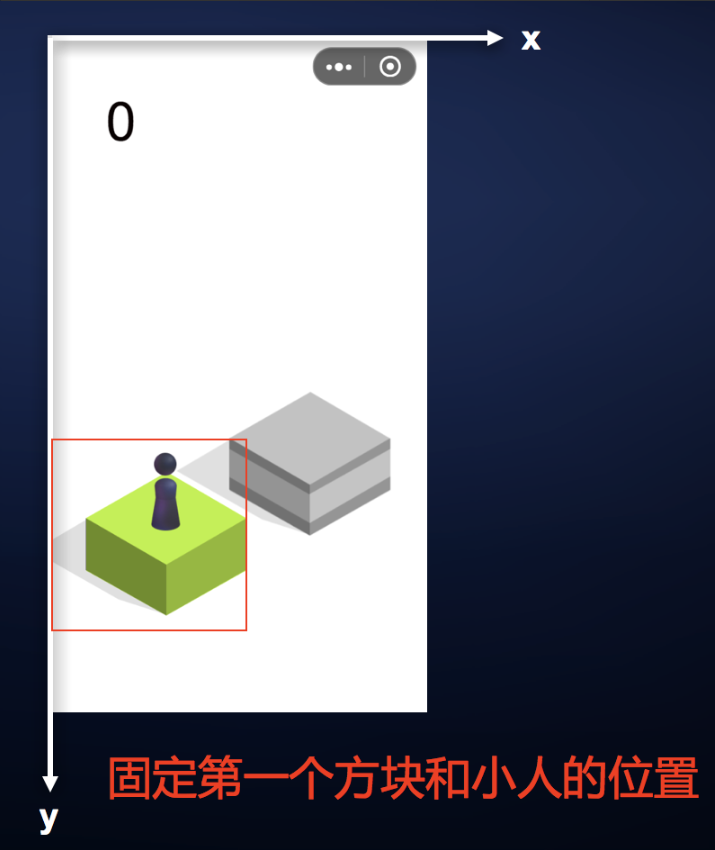ok，我们先简要看下一个方块是如何生成并添加到舞台上（重复的东西我们一般会写成一个类或者方法，这里写的是方法），其实就是贴图，好比我们用 `new Image()` 一样，再设置 `src` 等属性即可，具体请看下面代码：
``````// 创建一个方块
private createBlock(): eui.Image {✌
// 随机背景图
let n = Math.floor(Math.random() * this.blockSourceNames.length);
// 实例化并添加到舞台中
let blockNode = new eui.Image();
blockNode.source = this.blockSourceNames[n];
// 设置方块的锚点（之前说过的不是图片的中心点，而是图中盒子的中心点）
blockNode.anchorOffsetX = 222;
blockNode.anchorOffsetY = 78;
blockNode.touchEnabled = false;
// 把新创建的方块添加进入 blockArr 里，统一管理
this.blockArr.push(blockNode);
return blockNode;
}
// 添加一个方块并设置 xy 值
// 创建一个方块
let blockNode = this.createBlock();
// 随机水平位置（在最大最小值之间的一个数，毕竟屏幕就那么大）
let distance = this.minDistance + Math.random() * (this.maxDistance - this.minDistance);
if (this.direction > 0) { // 向右跳
blockNode.x = this.currentBlock.x + distance;
blockNode.y = this.currentBlock.y - distance * this.tanAngle;
} else { // 向左跳
blockNode.x = this.currentBlock.x - distance;
blockNode.y = this.currentBlock.y - distance * this.tanAngle;
}
this.currentBlock = blockNode;
}
``````

ok，现在我们知道了怎么创建方块，接下来就看看 `init` 函数里面的代码吧，瞅瞅初始化的时候都做了啥：

``````// SceneGame.ts
private init() {
// 所有盒子资源
this.blockSourceNames = ["block1_png", "block2_png", "block3_png"];
// 加载按下和跳跃的声音
this.pushVoice = RES.getRes('push_mp3');
this.jumpVoice = RES.getRes('jump_mp3');
// 初始化场景（方块和小人）
this.initBlock();
// 添加触摸事件
this.blockPanel.touchEnabled = true;
// 心跳计时器（目的：计算按的时长，推算出跳的距离）
egret.startTick(this.computeDistance, this);
}
private initBlock() {
// 初始化第一个方块，并设置相关的属性（主要就是在舞台中的位置也就是xy值）
this.currentBlock = this.createBlock();
this.currentBlock.x = this.leftOrigin.x;
this.currentBlock.y = this.stage.stageHeight - this.leftOrigin.y;
// 初始化小人（小人的锚点在底部的中间）
this.player.y = this.currentBlock.y;
this.player.x = this.currentBlock.x;
this.player.anchorOffsetX = this.player.width / 2;
this.player.anchorOffsetY = this.player.height - 20;
// 初始化得分
this.score = 0;
this.scoreLabel.text = this.score.toString();
// 初始化方向
this.direction = 1;
// 添加下一个盒子
}
``````

``````// SceneGame.ts
// 这个函数需要返回布尔值（规定），具体还不是很清楚它的作用，但不影响我们写代码
private computeDistance(timeStamp:number):boolean {
// timeStamp 是一个自增的时间（执行到当前所逝去的时间，比如0，500，1000...，单位 ms）
let now = timeStamp;
let time = this.time;
let pass = now - time;
pass /= 1000;
// 通过按压时间（就是 s = vt）来计算出跳的距离（这里指的是水平位移）
this.jumpDistance += 300 * pass; // 300 是调试出来的参数，可自行更改
}
this.time = now;
return true;
}
``````

### 起跳前

``````// SceneGame.ts
private onTapDown() {
// 播放按下音效，参数为（从哪里开始播放，播放次数）
this.pushSoundChannel = this.pushVoice.play(0, 1);
// 使小人变矮做出积蓄能量的效果，就是缩放Y轴
egret.Tween.get(this.player).to({scaleY: 0.5}, 3000);
// 起跳的标记
}
``````

### 起跳时

1、将舞台置为不可点击状态；
2、切换声音；
3、通过按压时间来计算跳跃的水平距离；
4、小人沿曲线起跳并旋转；

``````// SceneGame.ts
private onTapUp() {
if (!this.targetPos) this.targetPos = new egret.Point(); // point 就是个点，有 xy 值等

// 一松手小人就该起跳，此时应先禁止点击屏幕，并切换声音
this.blockPanel.touchEnabled = false;
this.pushSoundChannel.stop();
this.jumpVoice.play(0, 1);

// 清除所有动画
egret.Tween.removeAllTweens();

// 计算落点坐标
this.targetPos.x = this.player.x + this.direction * this.jumpDistance;
this.targetPos.y = this.player.y + this.direction * this.jumpDistance * (this.currentBlock.y - this.player.y) / (this.currentBlock.x - this.player.x);

// 执行跳跃动画
egret.Tween.get(this).to({ factor: 1 }, 400).call(() => { // 这表示贝塞尔曲线，在 400 毫秒内，this 的 factor 属性将会缓慢趋近1这个值，这里的 factor 就是曲线中的 t 属性，它是从 0 到 1 的闭区间。
this.player.scaleY = 1;
this.jumpDistance = 0;
// 判断跳跃是否成功
this.checkResult();
});
// 执行小人空翻动画，先处理旋转中心点
this.player.anchorOffsetY = this.player.height / 2;
egret.Tween.get(this.player)
.to({ rotation: this.direction > 0 ? 360 : -360 }, 200)
.call(() => { this.player.rotation = 0 })
.call(() => { this.player.anchorOffsetY = this.player.height - 20; });
}
// 添加 factor 的 set、get 方法
public get factor():number {
return 0;
}
// 这里的 getter 使 factor 属性从 0 开始，结合刚才 tween 中传入的 1，使其符合公式中的 t 的取值区间。
// 而重点是这里的 setter，里面的 player 对象是我们要应用二次贝塞尔曲线的显示对象，而在 setter 中给 player 对象的 xy 属性赋值的公式正是之前列出的二次贝塞尔曲线公式。
public set factor(t:number) {
// 仅仅是个公式
this.player.x = (1 - t) * (1 - t) * this.player.x + 2 * t * (1 - t) * (this.player.x + this.targetPos.x) / 2 + t * t * (this.targetPos.x);
this.player.y = (1 - t) * (1 - t) * this.player.y + 2 * t * (1 - t) * (this.targetPos.y - 300) + t * t * (this.targetPos.y);
}
``````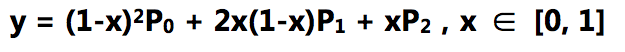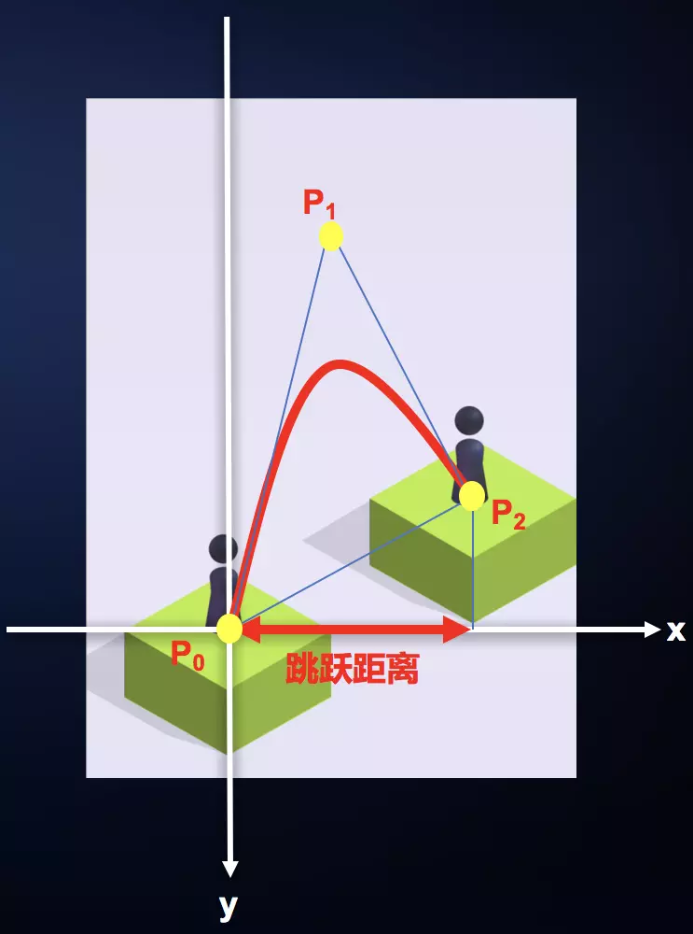``````egret.Tween.get(this.player).to({ x: this.targetPos.x, y: this.targetPos.y }, 400).call(() => {})
``````

### 起跳后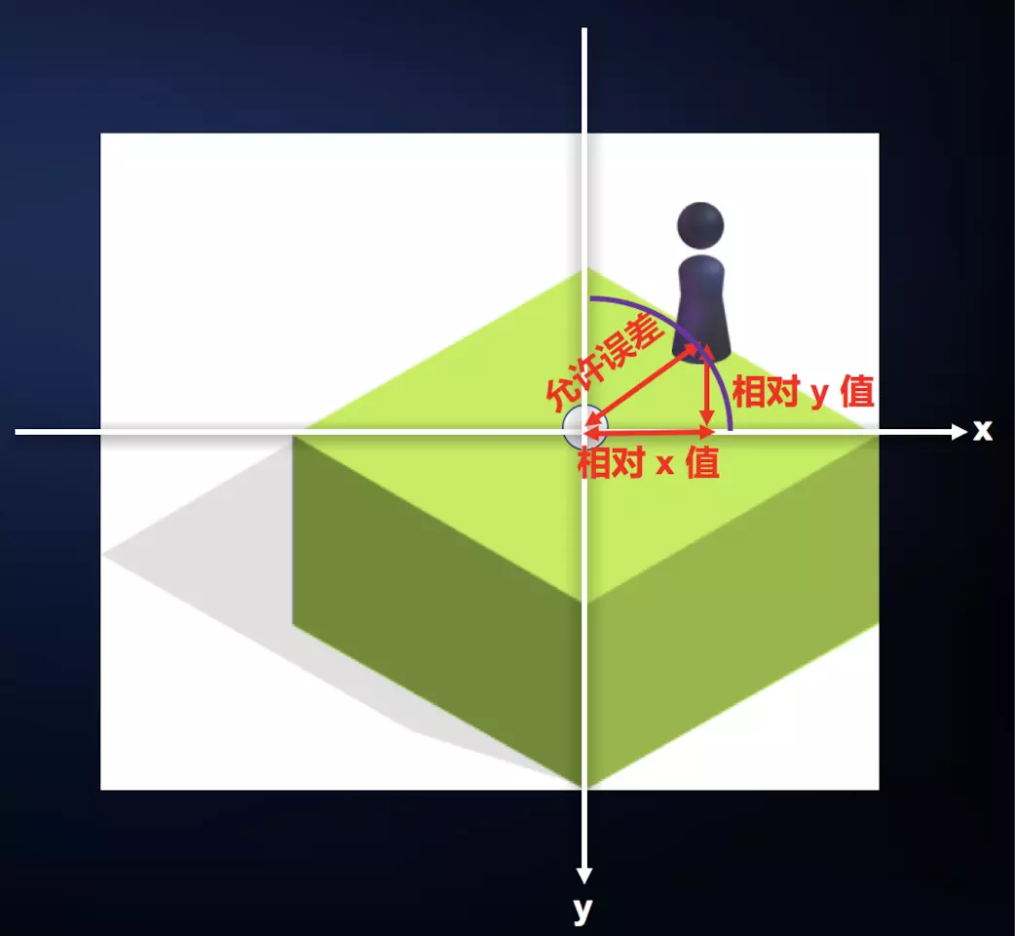``````// SceneGame.ts
private checkResult() {
// 实际误差
let err = Math.pow(this.player.x - this.currentBlock.x, 2) + Math.pow(this.player.y - this.currentBlock.y, 2)
// 允许的最大误差
const MAX_ERR_LEN = 90 * 90;
if (err <= MAX_ERR_LEN) { // 跳跃成功
// 更新分数
this.score++;
this.scoreLabel.text = this.score.toString();
// 要跳动的方向
this.direction = Math.random() > 0.5 ? 1 : -1;
// 当前方块要移动到相应跳跃点的距离
let blockX, blockY;
blockX = this.direction > 0 ? this.leftOrigin.x : this.rightOrigin.x;
blockY = this.stage.stageHeight / 2 + this.currentBlock.height;
// 小人要移动到的点
let diffX = this.currentBlock.x - blockX;
let diffY = this.currentBlock.y - blockY;
let playerX, playerY;
playerX = this.player.x - diffX;
playerY = this.player.y - diffY;
// 更新页面，更新所有方块位置
this.updateAll(diffX, diffY);
// 更新小人的位置
egret.Tween.get(this.player).to({
x: playerX,
y: playerY
}, 800).call(() => {
// 开始创建下一个方块
// 让屏幕重新可点;
this.blockPanel.touchEnabled = true;
})
} else { // 跳跃失败
this.overPanel.visible = true;
this.overScoreLabel.text = this.score.toString();
}
}
private updateAll(x, y) {
egret.Tween.removeAllTweens();
for (var i: number = this.blockArr.length - 1; i >= 0; i--) {
var blockNode = this.blockArr[i];
// 盒子的中心点（不是图片的中心点）在屏幕左侧 或者在 屏幕右侧 或者在 屏幕下方
if (blockNode.x + blockNode.width - 222 < 0 || blockNode.x - this.stage.stageWidth - 222 > 0 || blockNode.y - this.stage.stageHeight - 78 > 0) {
// 方块超出屏幕,从显示列表中移除
if (blockNode) this.blockPanel.removeChild(blockNode);
this.blockArr.splice(i, 1);
} else {
// 没有超出屏幕的话,则移动
egret.Tween.get(blockNode).to({
x: blockNode.x - x,
y: blockNode.y - y
}, 800)
}
}
}
``````

So 现在我们的首要任务就是知道其中一个方块怎么移，先来看看下面这张图：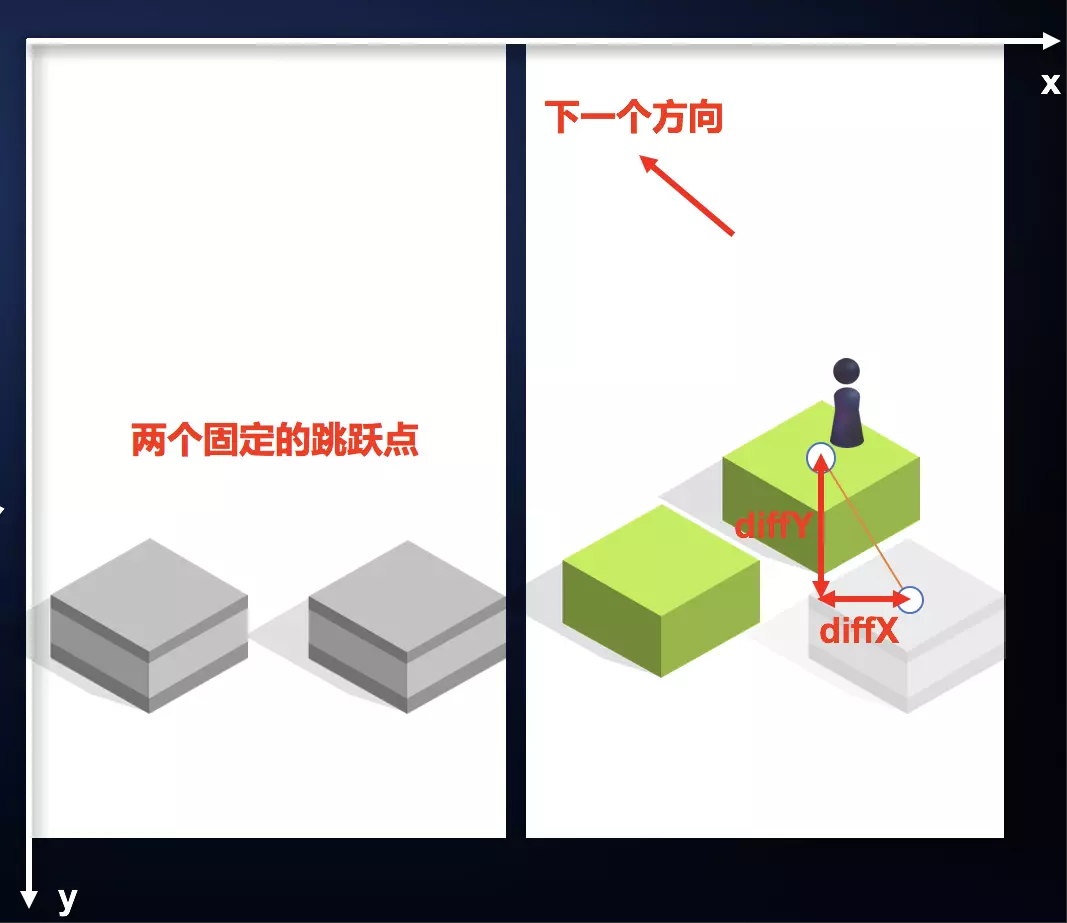## 运行游戏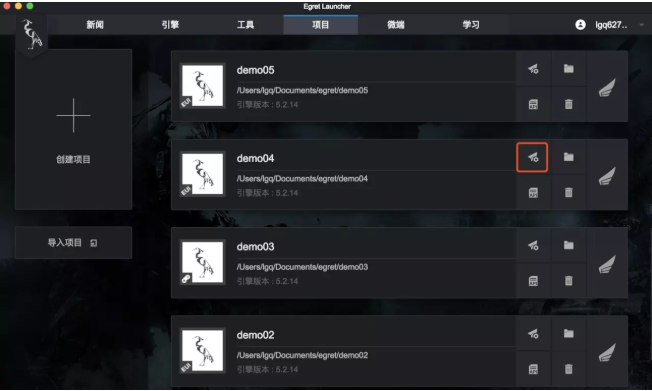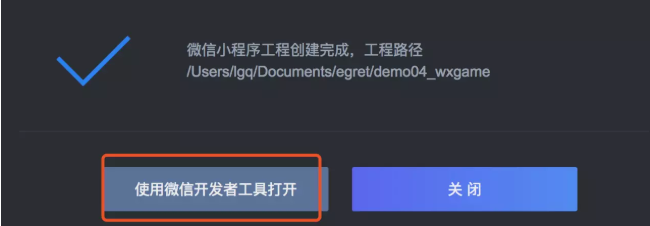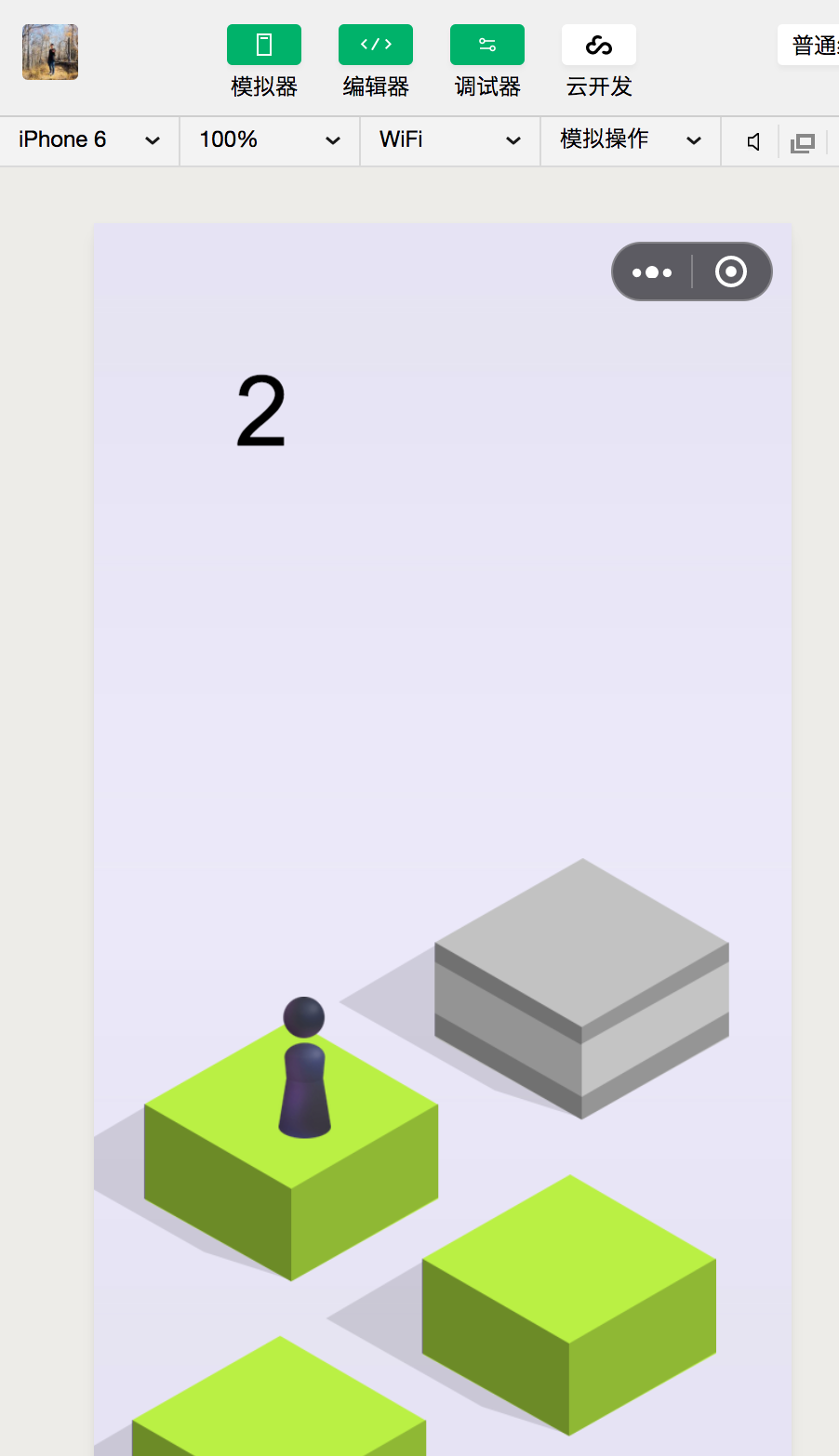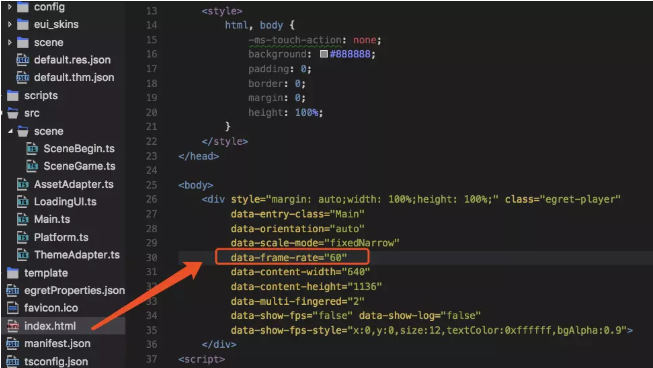## 结语

（转自掘金的egret 实战教程之跳一跳（二），作者：尤水就下也,链接:https://juejin.im/post/5c90b4175188252d7a5c4c15

## 标签# 相关推荐：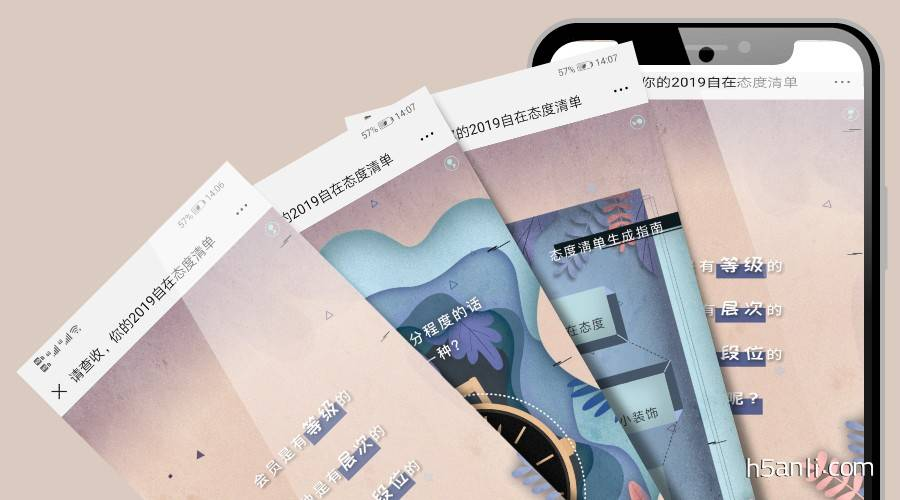## DANIEL WELLINGTON×京东闪购：请查收，你的2019自在态度清单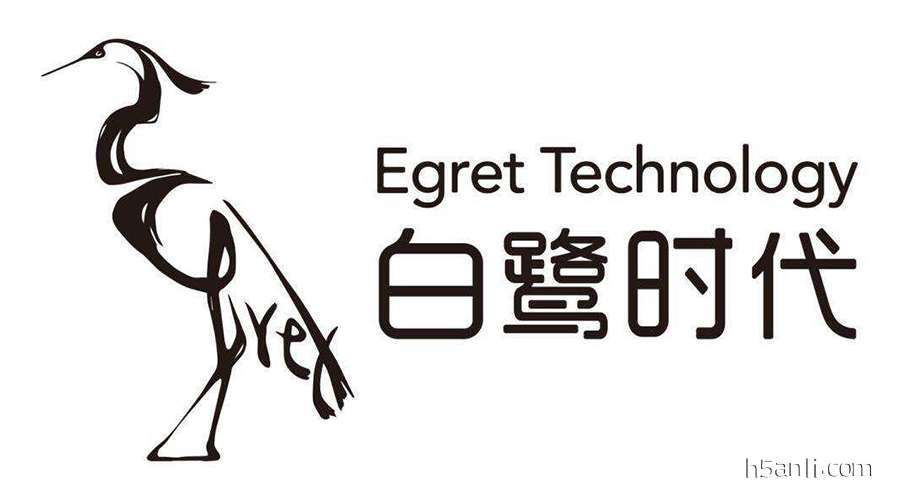## 掘金：egret 实战教程之跳一跳（一）## 10个案例了解H5之图片合成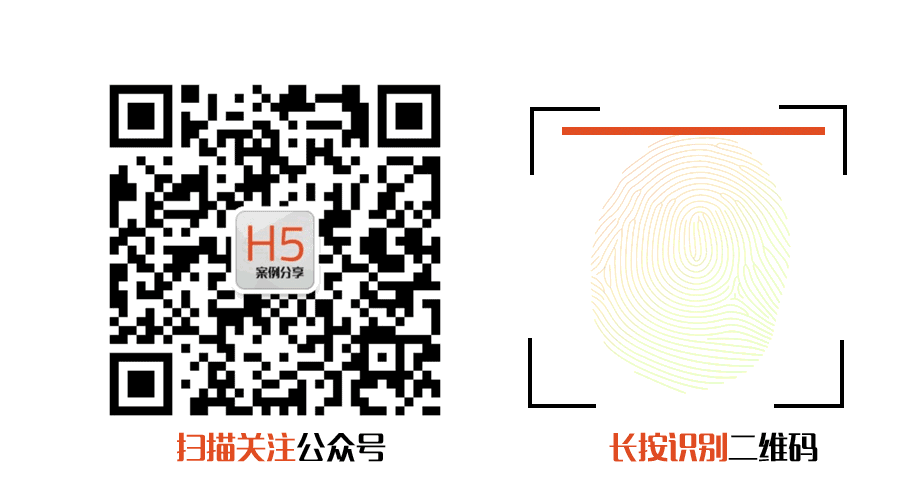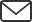#### 方式二："h5anli.com"